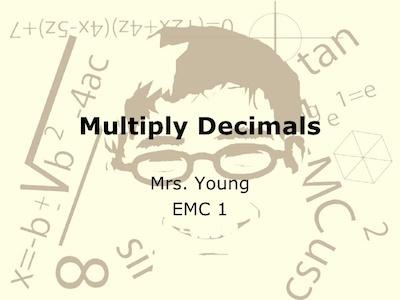# How To Multiply Decimals

When you are learning decimals, you need to know how to perform the simplest math operations such as multiply decimals.

The truth is that while i isn’t hard, many people struggle and don’t know exactly how to multiply decimals. So, today, we decided to show you exactly how you can do it easily as well as we’re going to show you some examples.

Learn how to round numbers up and down.

## How To Multiply Decimals

When you want to multiply decimals, you just need to follow the next steps:

Step #1: Multiply the numbers normally, ignoring the decimal points.

Step #2: Put the decimal point in the answer. It’s important to keep in mind that the answer will have as many decimal places as the two original numbers combined.

As you can easily understand, you just need to count up how many numbers are after the decimal point in both numbers you are multiplying, then the answer should have that many numbers after its decimal point.

Discover how to add and subtract decimals.

## Example: Multiply 0,03 By 1,1

0,03 × 1,1

Now, multiply without decimal points:

3 × 11 = 33

0,03 has 2 decimal places,

and 1,1 has 1 decimal place,

so the answer has 3 decimal places: 0,033

Discover how to convert unlike decimals to like decimals.

## How Did We Come Up With This?

When you multiply without the decimal point, you are really shifting the decimal point to the right to get it out of the way:

Original: 1 Move: 2 Moves: 3 Moves:

0,03 × 1,1 –>  0,3 × 1,1 –>   3. × 1,1 –>    3. × 11.

Then you do the multiplication:

3. × 11. = 33

But remember, you did 3 Moves of the decimal point, so you need to undo that:

3 Moves: 2 Moves: 1 Move: Correct

33. –>    3,3 –> 0,33       –>    0,033

Example: Multiply 0,25 By 0,2

0,25 × 0,2

Multiply without decimal points:

25 × 2 = 50

0,25 has 2 decimal places,

and 0,2 has 1 decimal place,

so the answer has 3 decimal places: 0,050

Example: Multiply 102 by 0,22

102 × 0,22

Multiply without decimal points:

102 × 22 = 2244

102 has 0 decimal places,

and 0,22 has 2 decimal place,

so the answer has 2 decimal places: 22,44

## How To Multiply A Decimal By A Whole Number

To multiply a decimal by a whole number, you just need to follow the below steps:

Step #1: Multiply the decimal without the decimal point by the whole number.

Step #2: Remember to mark the decimal point in the product from right to left according to the number of decimal places in the given decimal number.

Example #1: 5.36 × 8

Step #1: Find the product without the decimal point:

536 × 8 = 4288

Step #2: Since, 5.36 has two decimal places, 5.36 × 8 will also have two decimal places.

Therefore: 5.36 × 8 = 42.88

Example #2: 11.71 × 12

Step #1: Find the product without the decimal point:

1171 × 12 = 14052

Step #2: Since, 140.52 has two decimal places, 11.71 × 12 will also have two decimal places.

Therefore: 11.71 × 12 = 140.52